# Looking at Pictures topological analysis of patternsImage from Karl Sims.

## history of pattern analysis

Patterns exist on every scale; from a spiral galaxy down to the dendrites of a snowflake. There is no unifying theory to describe pattern formation but there are canonical ways to analyze.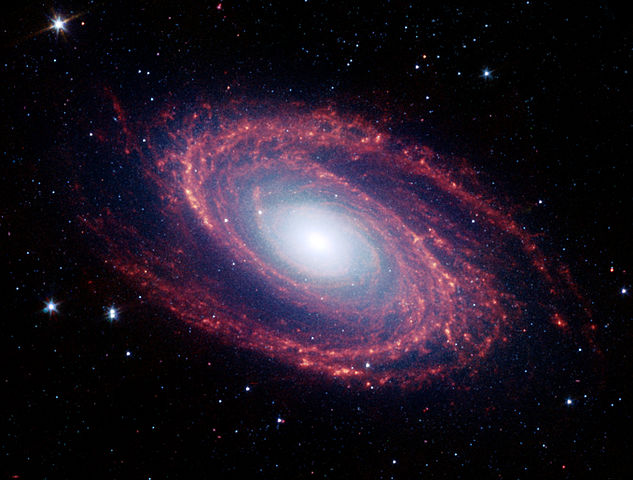M81 galaxy (NASA).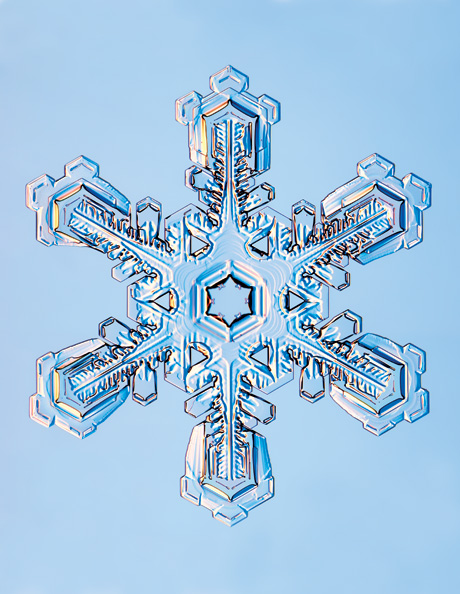Hexagonal snowflake dendrite (Kenneth Libbrecht).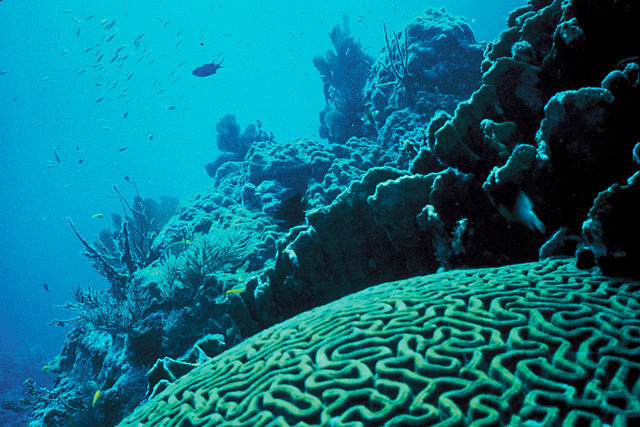Coral displays interesting pattern (U.S. Fish and Wildlife).

Rayleigh-Benard convection (Kurtuldu 2010).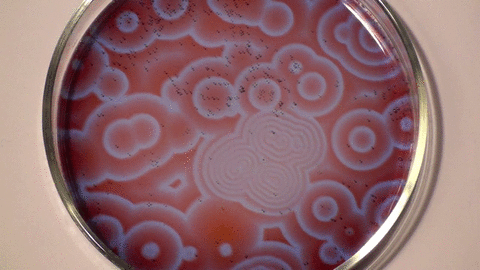Belousov–Zhabotinsky reaction (Stephen Morris, UofT).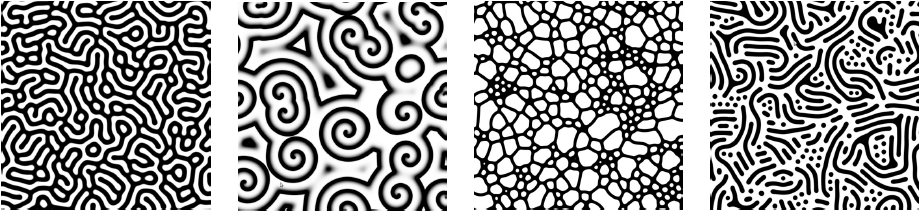Gray-Scott system (Karl Sims).

## methods of analysis

More data is great, but as the complexity of a system increases it becomes more difficult to extract relevant information.

The Fourier-Transform is a common method for pattern analysis, but for a complex pattern, it is difficult to draw conclusions from these methods → need new paradigms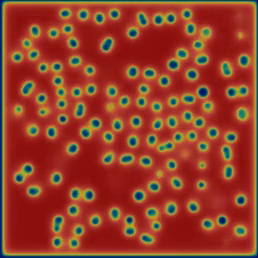Pattern alpha α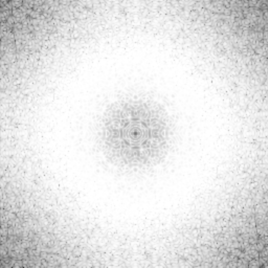Fast Fourier transform of alpha α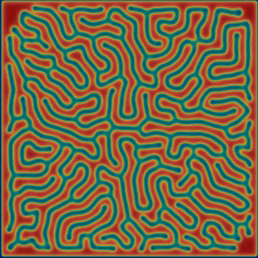Pattern kappa κ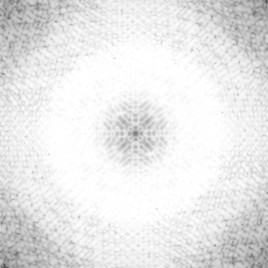Fast Fourier tranfsorm of kappa κ

## the gray-scott model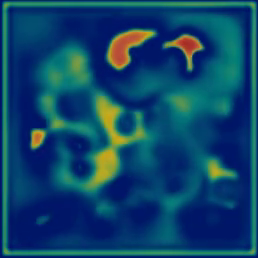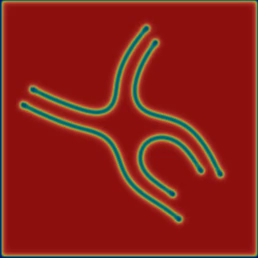### reaction diffusion systems

Reaction-diffusion (RD) systems determine how concentrations of substances change in space driven by two processes: chemical reaction and spatial diffusion. What makes them interesting is the wide variety of patterns they form and how many of those patterns resemble patterns of nature (e.g. spirals, stripes, and spots). In 1952, Alan Turing suggested that RD systems of morphogens may explain the presence of spots or stripes on an organism like a cheetah or zebra (Turing 1952). Turns out he was wrong but Turing laid the framework by which patterns form from minor perturbations of otherwise homogenous systems. Since then these types of systems have been studied extensively.

### the equations

Characterizes chemical interaction
U + 2V → 3V,
V → P.
Modeled by the following PDEs. $$\frac{\partial u}{\partial t} = d_u \nabla^2 u - uv^2 + F(1-u) \\ \frac{\partial v}{\partial t} = d_v \nabla^2 v + uv^2 - (F +k)v$$

• $\pm uv^2$ turns U into V
• $F(1-u)$ replenishes U
• $(F+k)v$ removes V from system
The Gray-Scott model is only toy system for us. The homology theory discussed later could be applied to any system!

## pattern types

For different (F, k), Pearson (1993) identified 14 different pattern types (see figure). The region near the bifurcation lines is the least stable and thus gives the most interesting patterns.

alpha exhibits spatio-temporal chaos. wavelets and "fledgeling-spirals" collide and annihilate upon hitting another.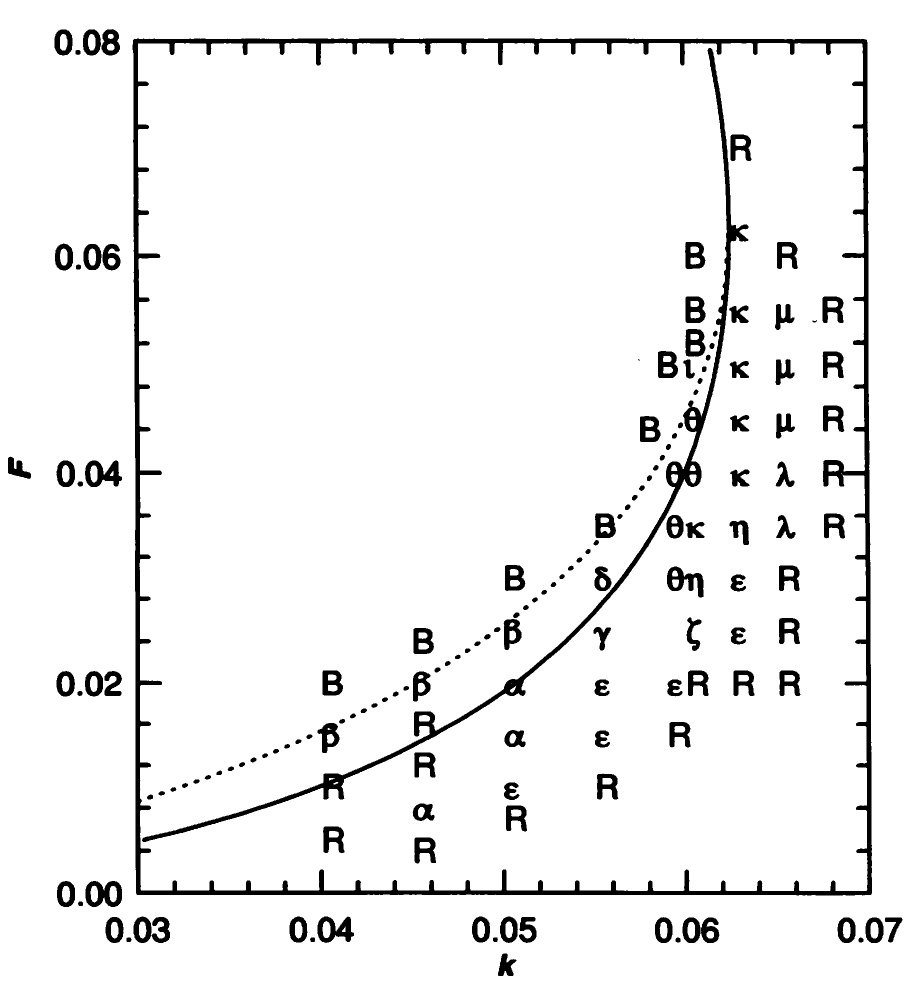A plot of (F,k) parameter space (Pearson 1993).

## computational homology

Homology is a method of extracting algebraic information from a topological space. This has the benefit of being extremely low-level by focusing on the geometric structures. We are primarily concerned with the Betti Numbers, or βi

• β0 is the # of connected pieces
• β1 is the # of holes (or loops)
• there are as many βi as dimensions of the space
• filled holes do not count!
In three dimensions (or higher), β2 represents the number of enclosed cavities (e.g. a bicycle tube has β0 = β1 = β2 = 1. ).

#### examples

β0 = 1
β1 = 1

β0 = 2
β1 = 1

β0 = 1
β1 = 0

β0 = 1
β1 = 1Homology of alpha α
β0 = 223
β1 = 1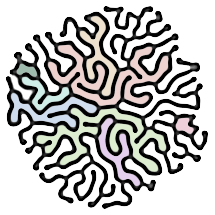Homology of kappa κ
β0 = 1
β1 = 9

## betti numbers

Choose a pattern below to see how the Betti numbers of a pattern change over time. The pairs {β01}i can be said to represent a 'state' of the system.

#### video

alpha exhibits spatio-temporal chaos. wavelets and "fledgeling-spirals" collide and annihilate upon hitting another.

β0 |
β1

Time steps

## calculating entropy

Given a state {β01}i, we can define

• Ni, # of times state i appears
• Pi, the probability of state Ni
• S, the Shannon entropy

$$S = - \sum_{i=1}^{N} P_i \log{P_i}.$$ S gives a measure of complexity of the system. Intuitively, it represents how easily we can predict the next state.

#### alpha α, S = 5.31233273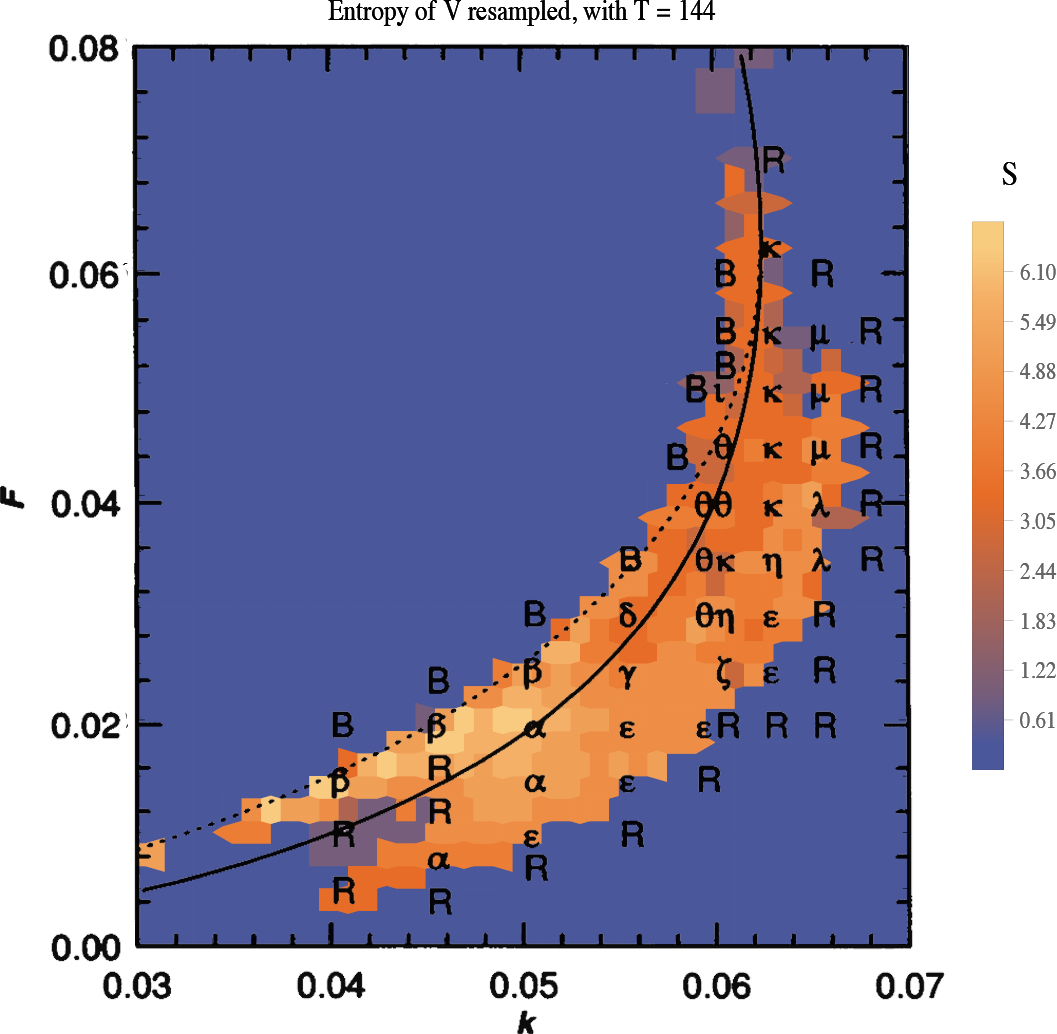The plot shows the entropy S over (F,k) parameter space.

How does the complexity information encoded in entropy relate to Pearson's data? Does it uncover more about the parameter space?
Open questions:

• entropy of 'natural' vs. 'unusual' patterns
• apply to non-simulated visual data

Homology is low-level analysis, but low-level geometric methods are the building blocks for higher levels of image analysis (e.g. fingerprint scanning, character recognization etc.).